PolarDB 数据库内核月报

# PolarDB 数据库内核月报 － 2016 / 11

## 背景

### 双十一背后的技术系列文章

《聊一聊双十一背后的技术 - 分词和搜索》

## 要素分析• 寄件人
• 揽件员
PS，揽件人通常不是直接将货收到仓库，而是网点。所以网点到仓库也是需要调度的，本文未涉及。
调度方法与配送差不太多。

• 货物
• 仓库
• 运输工具
• 派件员
• 收件人

### 1. 寄件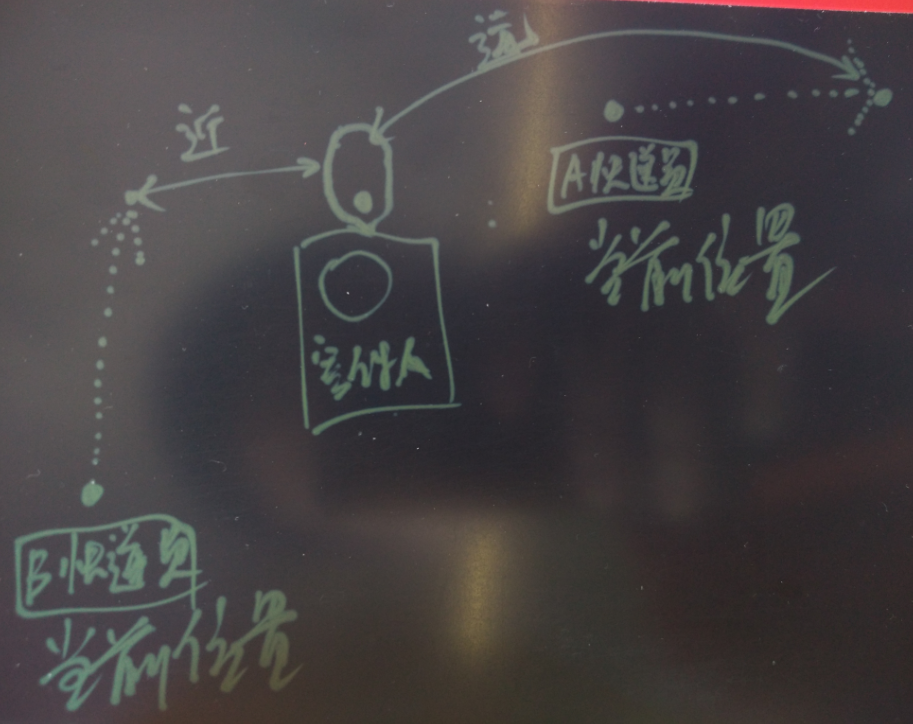### 2. 货物在仓库之间流转的物流调度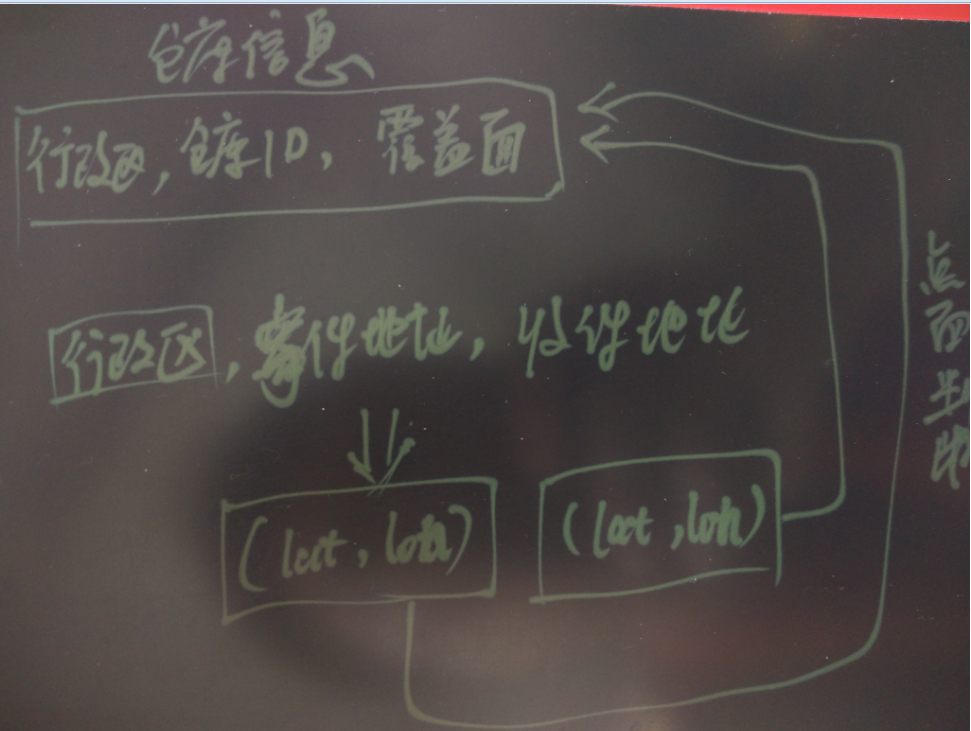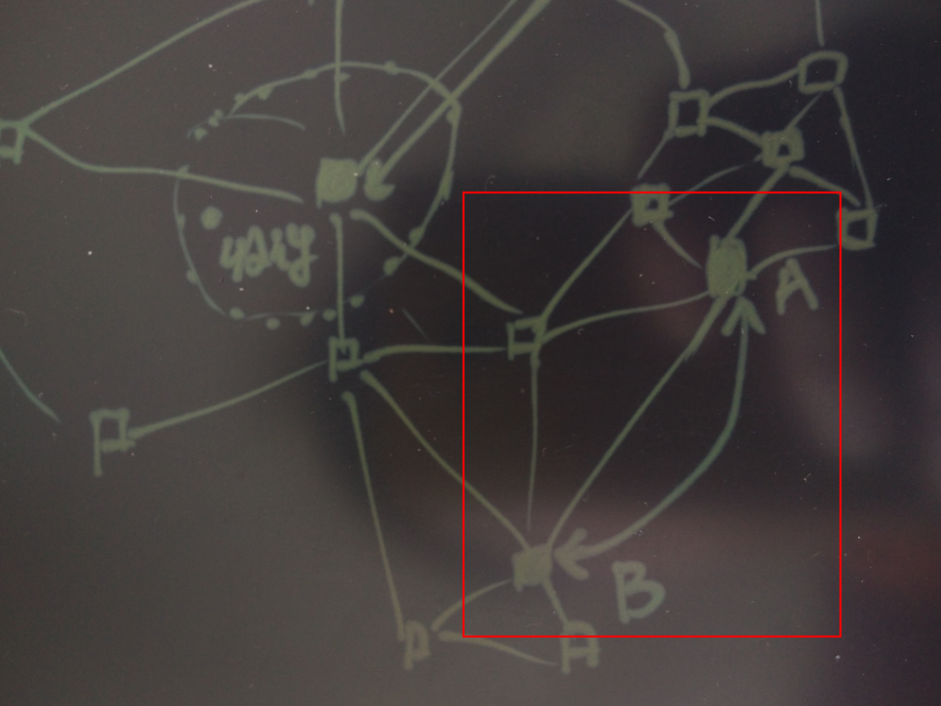### 4. 派件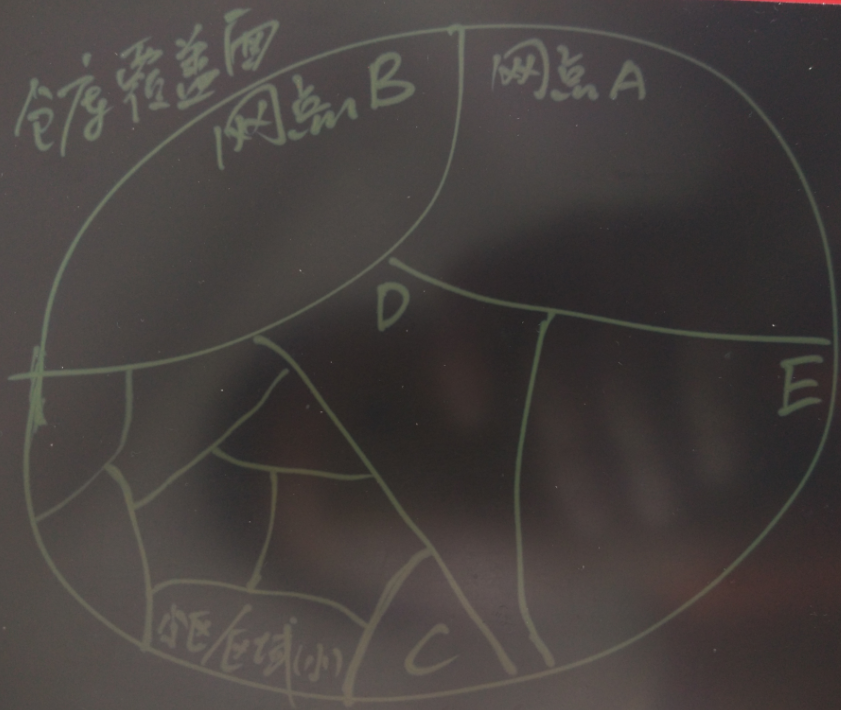## 最佳路径运算

``````Road link ID (gid)  唯一，指路段号
``````

``````               Table "public.ways"
Column  |           Type            | Modifiers
----------+---------------------------+-----------
gid      | bigint                    |
class_id | integer                   | not null
length   | double precision          |
name     | character(200)            |
osm_id   | bigint                    |
the_geom | geometry(LineString,4326) |
Indexes:
"ways_gid_idx" UNIQUE, btree (gid)
"geom_idx" gist (the_geom)
``````

### 1. 生成拓扑

``````-- Add "source" and "target" column
ALTER TABLE ways ADD COLUMN "source" integer;
ALTER TABLE ways ADD COLUMN "target" integer;
``````

``````pgr_createTopology(
'<table>',   -- 需要生成拓扑的表名
float tolerance,   --  容错值，例如线段的端不能完全吻合时，允许多少误差，单位一般为角度或公里数
'<geometry column>',   --  线段列名
'<gid>')  --  gid
``````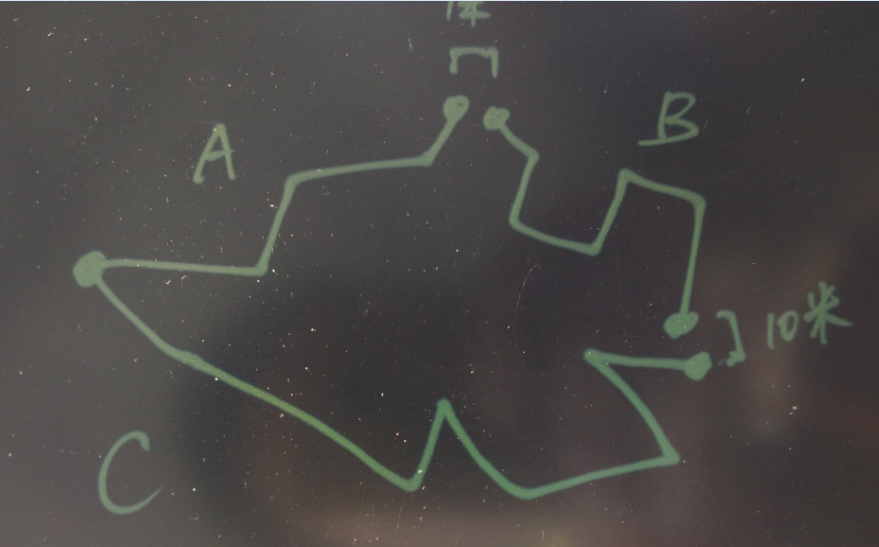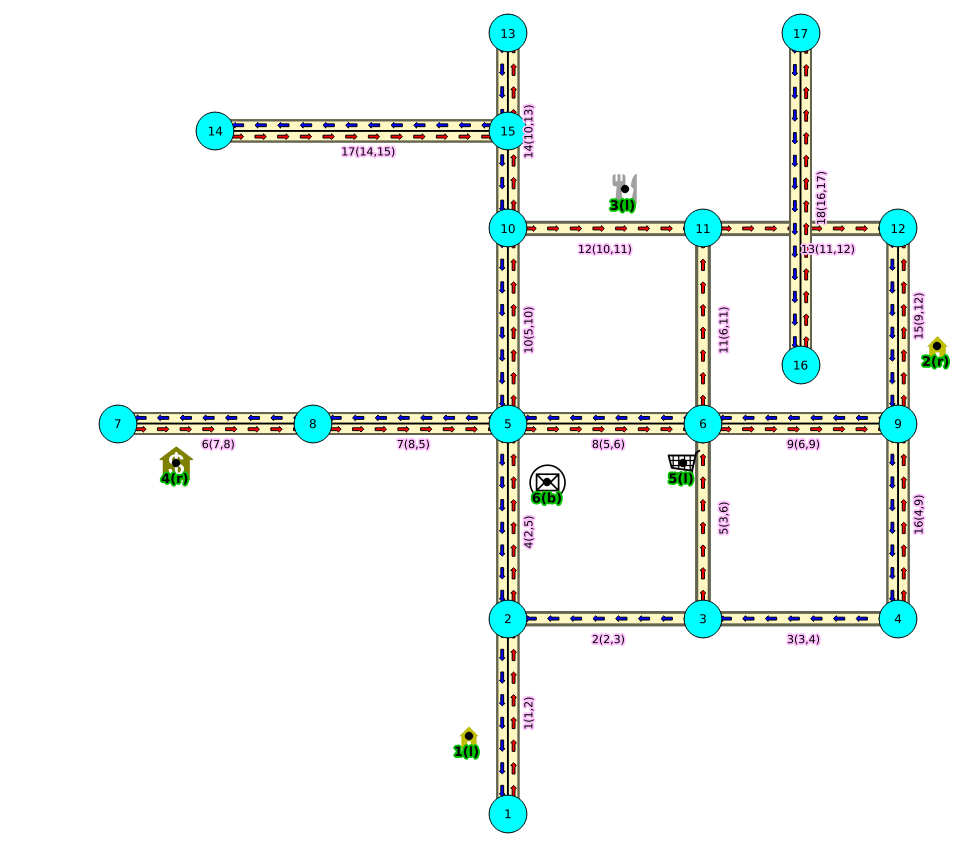``````-- Run topology function
SELECT pgr_createTopology('ways', 0.00001, 'the_geom', 'gid');
``````

### 2. 生成最佳路径

pgrouting支持的最佳路径算法很多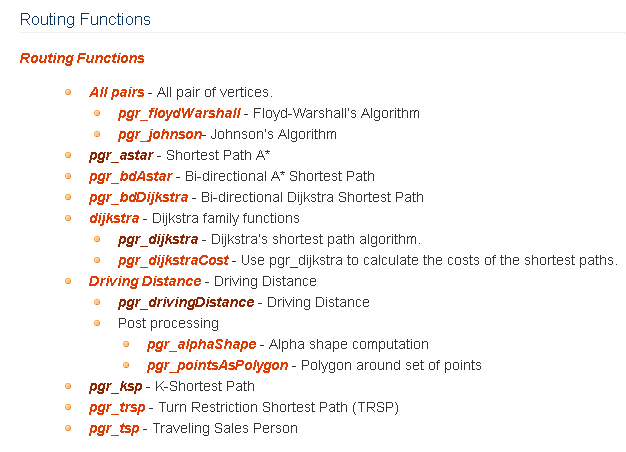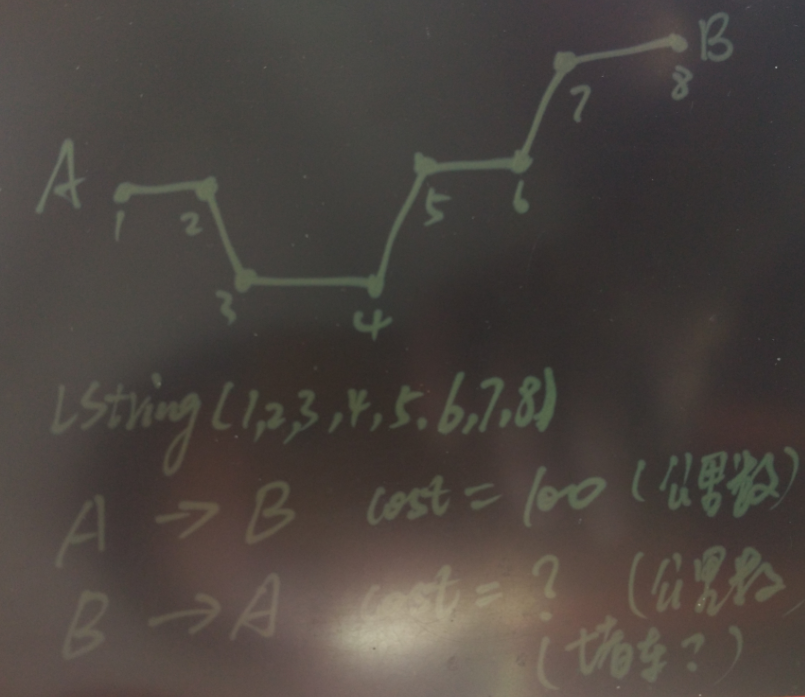``````ALTER TABLE ways ADD COLUMN reverse_cost double precision;
UPDATE ways SET reverse_cost = length;
``````

#### 2.1 Shortest Path Dijkstra算法举例

``````pgr_costResult[] pgr_dijkstra(
text sql, -- 用于计算最佳路径的数据来源, 用SQL表示, 例如
-- SELECT id (gid), source (线段起点id), target (线段重点ID), cost (起点到重点的成本) [,reverse_cost (重点到起点的成本)] FROM edge_table
integer source,   --  规划路径的起点
integer target,   --  规划路径的终点
boolean directed,   --  if the graph is directed
boolean has_rcost  -- if true, the reverse_cost column of the SQL generated set of rows will be used for the cost of the traversal of the edge in the opposite direction.
);
``````

``````a set of pgr_costResult (seq (序号), id1 (起点id), id2 (目标ID, -1表示终点), cost (这一段的成本)) rows, that make up a path.
``````

``````SELECT seq, id1 AS node, id2 AS edge, cost FROM pgr_dijkstra('
SELECT gid AS id,
source::integer,
target::integer,
length::double precision AS cost
FROM ways',
30, 60, false, false);

seq | node | edge |        cost
-----+------+------+---------------------
0 |   30 |   53 |  0.0591267653820616
1 |   44 |   52 |  0.0665408320949312
2 |   14 |   15 |  0.0809556879332114
...
6 |   10 | 6869 |  0.0164274192597773
7 |   59 |   72 |  0.0109385169537801
8 |   60 |   -1 |                   0
(9 rows)
``````

#### 2.2 Shortest Path A*算法举例

``````ALTER TABLE ways ADD COLUMN x1 double precision;
ALTER TABLE ways ADD COLUMN y1 double precision;
ALTER TABLE ways ADD COLUMN x2 double precision;
ALTER TABLE ways ADD COLUMN y2 double precision;

UPDATE ways SET x1 = ST_x(ST_PointN(the_geom, 1));  -- 线段起点坐标x
UPDATE ways SET y1 = ST_y(ST_PointN(the_geom, 1));  -- 线段起点坐标y

UPDATE ways SET x2 = ST_x(ST_PointN(the_geom, ST_NumPoints(the_geom)));  -- 线段终点坐标x
UPDATE ways SET y2 = ST_y(ST_PointN(the_geom, ST_NumPoints(the_geom)));  -- 线段终点坐标y
``````

``````pgr_costResult[] pgr_astar(
sql text,     -- SELECT id, source, target, cost, x1, y1, x2, y2 [,reverse_cost] FROM edge_table ，包含了起点和重点坐标，计算速度比Shortest Path A*算法快一点
source integer,
target integer,
directed boolean,
has_rcost boolean
);
``````

``````a set of pgr_costResult (seq, id1, id2, cost) rows, that make up a path.
``````

``````SELECT seq, id1 AS node, id2 AS edge, cost FROM pgr_astar('
SELECT gid AS id,
source::integer,
target::integer,
length::double precision AS cost,
x1, y1, x2, y2
FROM ways',
30, 60, false, false);
``````

`````` seq | node | edge |        cost
-----+------+------+---------------------
0 |   30 |   53 |  0.0591267653820616
1 |   44 |   52 |  0.0665408320949312
2 |   14 |   15 |  0.0809556879332114
...
6 |   10 | 6869 |  0.0164274192597773
7 |   59 |   72 |  0.0109385169537801
8 |   60 |   -1 |                   0
(9 rows)
``````

#### 2.3 生成多目标最佳路径

##### 生成分段成本
``````pgr_costResult[] pgr_kdijkstraCost(text sql, integer source,
integer[] targets, boolean directed, boolean has_rcost);
``````

``````SELECT seq, id1 AS source, id2 AS target, cost FROM pgr_kdijkstraCost('
SELECT gid AS id,
source::integer,
target::integer,
length::double precision AS cost
FROM ways',
10, array[60,70,80], false, false);

seq | source | target |       cost
-----+--------+--------+------------------
0 |     10 |     60 | 13.4770181770774
1 |     10 |     70 | 16.9231630493294
2 |     10 |     80 | 17.7035050077573
(3 rows)
``````
##### 生成路径
``````pgr_costResult[] pgr_kdijkstraPath(text sql, integer source,
integer[] targets, boolean directed, boolean has_rcost);
``````

``````SELECT seq, id1 AS path, id2 AS edge, cost FROM pgr_kdijkstraPath('
SELECT gid AS id,
source::integer,
target::integer,
length::double precision AS cost
FROM ways',
10, array[60,70,80], false, false);

seq | path | edge |        cost
-----+------+------+---------------------
0 |   60 | 3163 |   0.427103399132954
1 |   60 | 2098 |   0.441091435851107
...
40 |   60 |   56 |  0.0452819891352444
41 |   70 | 3163 |   0.427103399132954
42 |   70 | 2098 |   0.441091435851107
...
147 |   80 |  226 |  0.0730263299529259
148 |   80 |  227 |  0.0741906229622583
(149 rows)
``````

## 小结

1. 点面判断

2. 点面判断后，按面进行聚合

3. 路径规划

4. 支持哪些最优算法

5. UDF

PostgreSQL支持python, R, C等各种自定义函数的语言

6. 机器学习

7. 地址转换成坐标

8. 聚类算法

``````    SELECT kmeans(ARRAY[x, y, z], 10) OVER (), * FROM samples;
SELECT kmeans(ARRAY[x, y], 2, ARRAY[0.5, 0.5, 1.0, 1.0]) OVER (), * FROM samples;
SELECT kmeans(ARRAY[x, y, z], 2, ARRAY[ARRAY[0.5, 0.5], ARRAY[1.0, 1.0]]) OVER (PARTITION BY group_key), * FROM samples;
``````

PostgreSQL在地理位置处理的领域一直处于非常领先的地位，用户群体也非常的庞大，PostGIS和pgrouting只是这个领域的两插件。

PostGIS和pgrouting在阿里云的RDS PG数据库都有提供，欢迎使用。